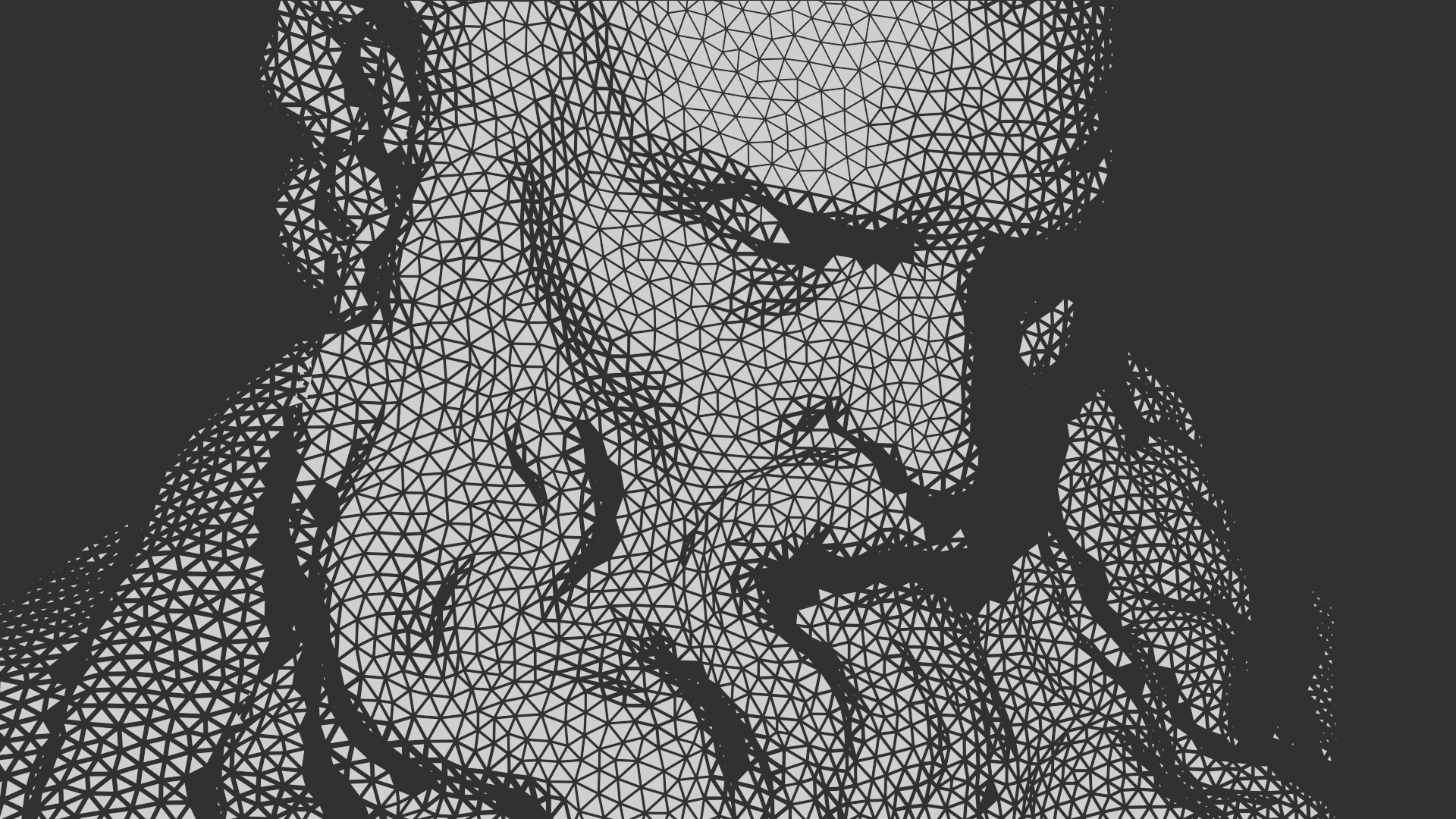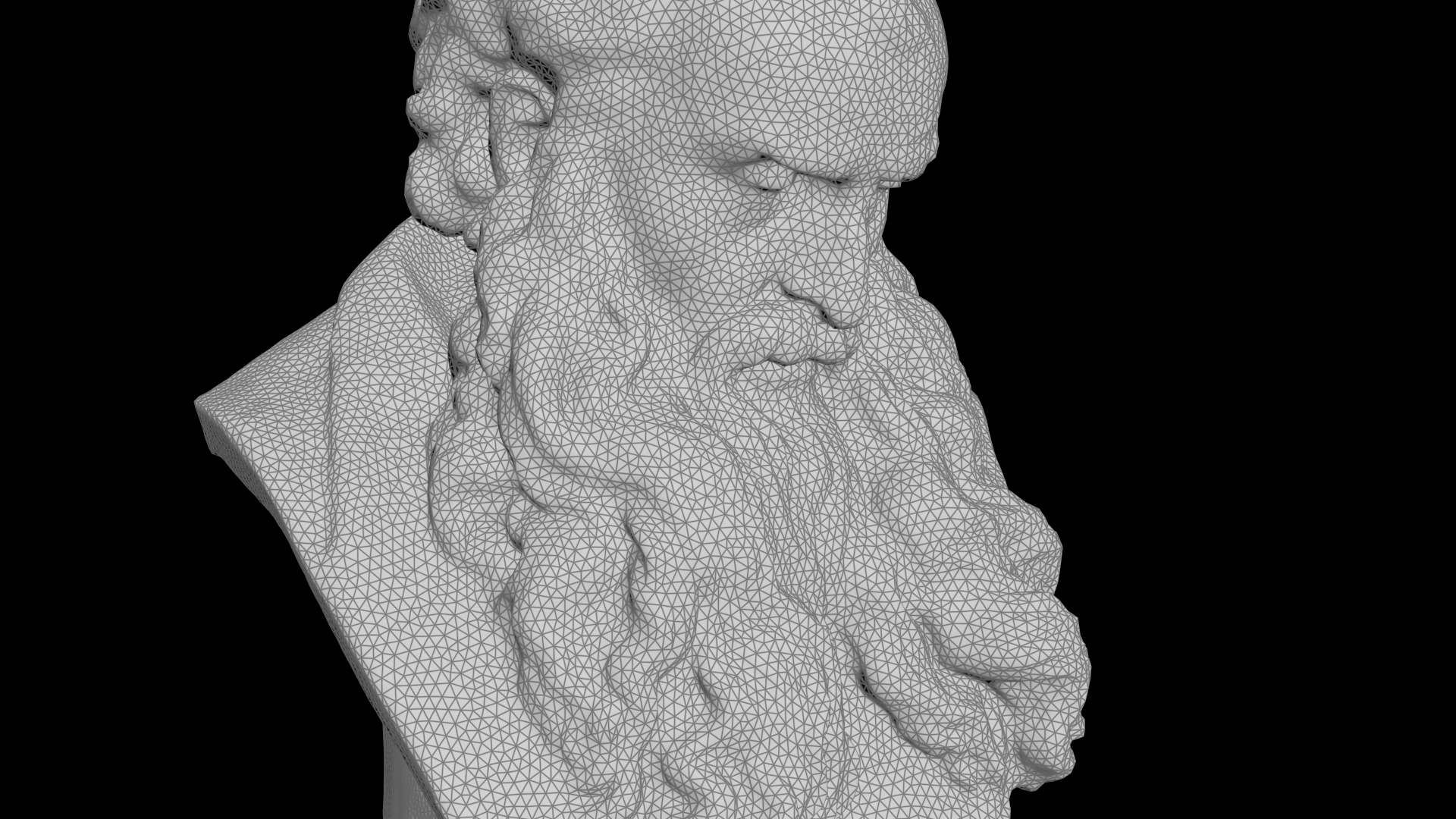# Lighting 2D triangles from a 3D mesh

calendar_today

01.05.2022

label

VEX, Rendering

mouse

Houdini 19.0

### 1 IntroductionLet's display a triangulated 3D mesh as a lit and shaded perspective view in the form of 2D triangles. This supports the cameras perspective including removal of backface polygons as well as shading and lighting with attenuation and raytraced shadows.

### 2 Process

First we integrate the parameters for the camera and light operator path along with an intensity slider. Based on this we'll extract the camera perspective and light directions which are then being used for shading/lighting as well as raycasting each point towards the camera and the light. We'll remove the points if any of their rays intersect with the mesh indicating they are either not seen and/or not lit. Lastly, we transform the point positions into camera space and, based on our lighting calculation, output an `inset` attribute to be used later in a poly extrude node.

### 3 Code

``````// PARAMETERS
string cam = chs('camera');
string light = chs('light');
float intens = chf('intensity');

// PERSPECTIVE
vector pos_near = fromNDC(cam, {0.5, 0.5, 0.0});
vector pos_far = fromNDC(cam, {0.5, 0.5, -0.1});
vector dir_cam = normalize(pos_near - pos_far);
vector pos = toNDC(cam, v@P);
pos.y *= 9.0/16.0;

// LIGHTING
matrix xform_light = optransform(light);
vector pos_light = cracktransform(0, 0, 0, 0, 0, xform_light);
vector dir_light = normalize(pos_light - v@P);

vector nml = normalize(v@N);
float atten = intens - distance2(v@P, pos_light);
float lighting = dot(nml, dir_light);
float shading = max(dot(nml, dir_cam), 0.0);
float inset = min(atten, lighting, shading);

// RAYCASTING
vector pos_srf = v@P + nml * 1e-3;
int prim_cam = intersect(0, pos_srf, dir_cam, set(0), set(0));
int prim_light = intersect(0, pos_srf, dir_light, set(0), set(0));
if(prim_cam + prim_light >= 0) removepoint(0, i@ptnum, 0);

// ATTRIBUTES
v@P = set(pos.x, pos.y, 0.0);
f@inset = fit01(inset, 0.0025, 0.0);``````